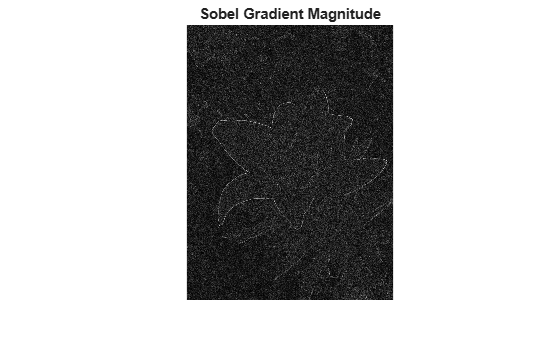# Reduce Noise in Image Gradients

This example demonstrates how to reduce noise associated with computing image gradients. Image gradients are used to highlight interesting features in images and are used in many feature detection algorithms like edge/corner detection. Reducing noise in gradient computations is crucial to detecting accurate features.

Read an image into the workspace and convert it to grayscale.

```originalImage = imread('yellowlily.jpg'); originalImage = rgb2gray(originalImage); imshow(originalImage)```To simulate noise for this example, add some Gaussian noise to the image.

```noisyImage = imnoise(originalImage,'gaussian'); imshow(noisyImage)```Compute the magnitude of the gradient by using the `imgradient` and `imgradientxy` functions. `imgradient` finds the gradient magnitude and direction, and `imgradientxy` finds directional image gradients.

```sobelGradient = imgradient(noisyImage); imshow(sobelGradient,[]) title('Sobel Gradient Magnitude')```Looking at the gradient magnitude image, it is clear that the image gradient is very noisy. The effect of noise can be minimized by smoothing before gradient computation. `imgradient` already offers this capability for small amounts of noise by using the Sobel gradient operator. The Sobel gradient operators are 3x3 filters as shown below. They can be generated using the `fspecial` function.

`hy = -fspecial('sobel')`
```hy = 3×3 -1 -2 -1 0 0 0 1 2 1 ```
`hx = hy'`
```hx = 3×3 -1 0 1 -2 0 2 -1 0 1 ```

The `hy` filter computes a gradient along the vertical direction while smoothing in the horizontal direction. `hx` smooths in the vertical direction and computes a gradient along the horizontal direction. The `'Prewitt'` and `'Roberts'` method options also provide this capability.

Even with the use of Sobel, Roberts or Prewitt gradient operators, the image gradient may be too noisy. To overcome this, smooth the image using a Gaussian smoothing filter before computing image gradients. Use the `imgaussfilt` function to smooth the image. The standard deviation of the Gaussian filter varies the extent of smoothing. Since smoothing is taken care of by Gaussian filtering, the central or intermediate differencing gradient operators can be used.

```sigma = 2; smoothImage = imgaussfilt(noisyImage,sigma); smoothGradient = imgradient(smoothImage,'CentralDifference'); imshow(smoothGradient,[]) title('Smoothed Gradient Magnitude')```